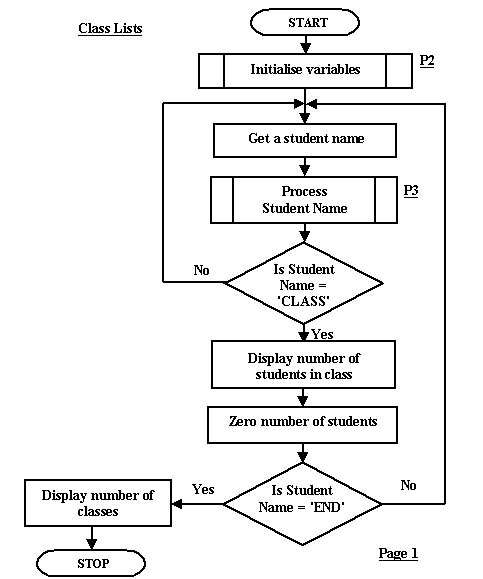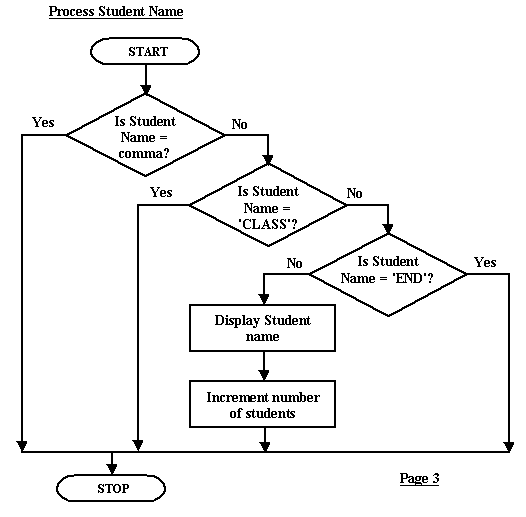### Flow charts: An advanced flow chart - Exercise 3

Assume you have the following data stored somewhere:

Fred,Joan,Brian,Bert,Selie,Sue,Jack,Ng,Jacques,CLASS,Chris,Cheryl,Pam,Allan,CLASS,END

and it represents students in different classes.

Design a program using flow charts which:

1. reads the data and displays the names of the students in the class
2. counts the number of students in each class
3. counts the number of classes

Here is the pseudocode I offered as a sample answer before:

Students_In_Class = 0
Number_Of_Classes = 0
REPEAT
REPEAT
INPUT Student_Name
IF Student_Name <> ','
THEN IF Student_Name <> 'CLASS'
THEN IF Student_Name <> 'END'
THEN  DISPLAY Student_Name
Students_In_Class = Students_In_Class + 1
ENDIF
UNTIL Student_Name = 'CLASS'
Number_Of_Classes = Number_Of_Classes + 1
DISPLAY 'Number of students is' Students_In_Class
Students_In_Class = 0
UNTIL Student_Name = 'END'
DISPLAY Number_Of_Classes

I've taken this and changed it to a flow chart but I've included subprocesses:The main flow chart contains two subprocesses, one to initialise the variables and the other to process the input data. I've the second one below but haven't bothered with the initialisation subprocess. You can see the nested repeat loops both returning to the same point of the flow chart, the entry point to the get student name process. The equivalent point in the pseudocode is the start of the two repeat statements.The subprocess here depicts multiway selection. In this case we have data that can be a student name or it can be one of three kinds of boundary value: a comma - the boundary between student names, a 'CLASS' word - the boundary between classes, a 'END' word - the end of the data.

and may not be reproduced by any means without the written

This publication is copyright David Beech and Learning Systems 1997-2002
and may not be reproduced by any means without the written permission of
David Beech.
9 Wyndella Street, Tasmania, Australia

db@codelearn.com